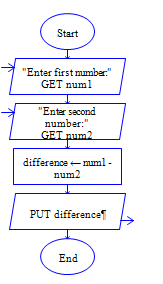# Java Program to subtraction of two numbers

Get two integer numbers, subtract both the integers and display the difference

Sample Input 1:

6 5

Sample Output 1:

-1

Sample Input 2:

65 4

Sample Output 2:

61

#### Flow Chart Design#### Program or Solution

``` import java.util.*; class Subtraction { public static void main(String args[]) { int Input1,Input2,difference; Scanner sc=new Scanner(System.in); System.out.println("Enter The Input 1:"); Input1=sc.nextInt(); System.out.println("Enter The Input 2:"); Input2=sc.nextInt(); difference=Input1-Input2; System.out.println("The Output Is:\n"+ difference); } } ```

#### Program Explanation

Get two integers Input1 and Input2 (using scanner class) Subract Input2 from Input1, then store the difference in sub (sub= Input1-Input2) print the value of sub (using system.out.println)